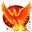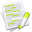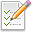#wx.Point2D¶

##Class Hierarchy¶Inheritance diagram for class Point2D:

##Methods Summary¶

 __init__ Get Get() . (x,y) GetCrossProduct GetDistance GetDistanceSquare GetDotProduct GetFloor GetIM Returns an immutable representation of the wx.Point2D object, based on namedtuple. GetRounded GetVectorAngle GetVectorLength Normalize SetVectorAngle SetVectorLength __bool__ __getitem__ __len__ __nonzero__ __reduce__ __repr__ __setitem__ __str__ __ne__ __imul__ __iadd__ __sub__ __isub__ __idiv__ __eq__

##Properties Summary¶

 IM VectorAngle VectorLength m_x A public C++ attribute of type Double . m_y A public C++ attribute of type Double .

##Class API¶

class wx.Point2D(object)

Possible constructors:

Point2DDouble()

Point2DDouble(x, y)

Point2DDouble(pt)

Point2DDouble(pt)


### Methods¶

__init__(self, *args, **kw)Overloaded Implementations:

__init__ (self)

__init__ (self, x, y)

Parameters
• x (wx.Double) –

• y (wx.Double) –

__init__ (self, pt)

Parameters

pt (Point2DDouble) –

__init__ (self, pt)

Parameters

pt (wx.Point) –

Get(self)

Get() . (x,y)

Return the x and y properties as a tuple.

Return type

PyObject

GetCrossProduct(self, vec)
Parameters

vec (Point2DDouble) –

Return type

wx.Double

GetDistance(self, pt)
Parameters

pt (Point2DDouble) –

Return type

wx.Double

GetDistanceSquare(self, pt)
Parameters

pt (Point2DDouble) –

Return type

wx.Double

GetDotProduct(self, vec)
Parameters

vec (Point2DDouble) –

Return type

wx.Double

GetFloor(self)
Return type

tuple

Returns

( x, y )

GetIM(self)

Returns an immutable representation of the wx.Point2D object, based on namedtuple.

This new object is hashable and can be used as a dictionary key, be added to sets, etc. It can be converted back into a real wx.Point2D with a simple statement like this: obj = wx.Point2D(imObj).

GetRounded(self)
Return type

tuple

Returns

( x, y )

GetVectorAngle(self)
Return type

wx.Double

GetVectorLength(self)
Return type

wx.Double

Normalize(self)

SetVectorAngle(self, degrees)
Parameters

degrees (wx.Double) –

SetVectorLength(self, length)
Parameters

length (wx.Double) –

__bool__(self)

__getitem__(self, idx)

__len__(self)

__nonzero__(self)

__reduce__(self)

__repr__(self)

__setitem__(self, idx, val)

__str__(self)

__ne__(self)
Parameters

pt (Point2DDouble) –

__imul__(self)
Parameters

pt (Point2DDouble) –

__iadd__(self)
Parameters

pt (Point2DDouble) –

__sub__(self)

__isub__(self)
Parameters

pt (Point2DDouble) –

__idiv__(self)
Parameters

pt (Point2DDouble) –

__eq__(self)
Parameters

pt (Point2DDouble) –

### Properties¶

IM

VectorAngle

VectorLength

m_x

A public C++ attribute of type Double .

m_y

A public C++ attribute of type Double .